Search

Conics: Ellipses:
Finding the Equation from Information
(page 3 of 4)

Sections: Introduction, Finding information from the equation, Finding the equation from information, Word ProblemsYou'll also need to work the other way, finding the equation for an ellipse from a list of its properties.

• Write an equation for the ellipse having one focus at (0, 3), a vertex at (0, 4),
and its center at
(0, 0).
• Since the focus and vertex are above and below each other, rather than side by side, I know that this ellipse must be taller than it is wide. Then a2 will go with the y part of the equation. Also, since the focus is 3 units above the center, then c = 3; since the vertex is 4 units above, then a = 4. The equation b2 = a2  c2 gives me 16  9 = 7 = b2. (Since I wasn't asked for the length of the minor axis or the location of the co-vertices, I don't need the value of b itself.) Then my equation is: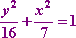• Write an equation for the ellipse with vertices (4, 0) and (2, 0)
and foci
• The center is midway between the two foci, so (h, k) = (1, 0), by the Midpoint Formula. Each focus is 2 units from the center, so c = 2. The vertices are 3 units from the center, so a = 3. Also, the foci and vertices are to the left and right of each other, so this ellipse is wider than it is tall, and a2 will go with the x part of the ellipse equation.

The equation b2 = a2  c2 gives me 9  4 = 5 = b2, and this is all I need to create my equation: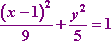• Write an equation for the ellipse centered at the origin, having a vertex at (0, 5) and containing the point (2, 4).
• Since the vertex is 5 units below the center, then this vertex is taller than it is wide, and the a2 will go with the y part of the equation. Also, a = 5, so a2 = 25. I know that b2 = a2  c2, but I don't know the values of b or c. However, I do have the values of h, k, and a, and also a set of values for x and y, those values being the point they gave me on the ellipse. So I'll set up the equation with everything I've got so far, and solve for b.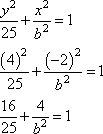16b2 + 100 = 25b2
100 = 9b2

100/9 = b2

Then my equation is: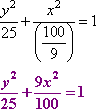• Write an equation for the ellipse having foci at (2, 0) and (2, 0)
and eccentricity
e = 3/4.
• The center is between the two foci, so (h, k) = (0, 0). Since the foci are 2 units to either side of the center, then c = 2, this ellipse is wider than it is tall, and a2 will go with the x part of the equation. I know that e = c/a, so 3/4 = 2/a. Solving the proportion, I get a = 8/3, so a2 = 64/9. The equation b2 = a2  c2 gives me 64/9  4 = 64/9  36/9 = 28/9 = b2.

Now that I have values for a2 and b2, I can create my equation: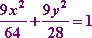<< Previous  Top  |  1 | 2 | 3 | 4  |  Return to Index  Next >>

 Cite this article as: Stapel, Elizabeth. "Conics: Ellipses: Finding the Equation from Information." Purplemath.     Available from https://www.purplemath.com/modules/ellipse3.htm.     Accessed [Date] [Month] 2016

Study Skills Survey

Tutoring from Purplemath
Find a local math tutor